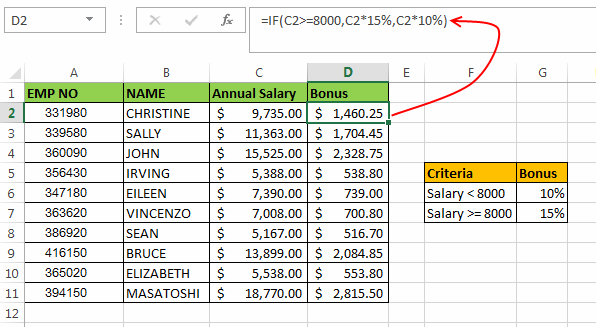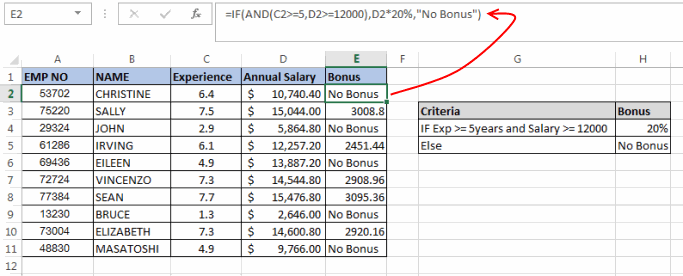# Excel IF Statement – How to Use

IF Statement is one of the most popular instructions among the Decision Making statements. IF Statement gives the desired intelligence to a program, so that it can take decisions based on a criteria and most importantly decide the program flow.

In Microsoft Excel, IF Statements can be quite useful for evaluating an expression (expressions can be both Mathematical as well as Logical) and giving an output based on it.

### Definition of IF Instruction:

According to Microsoft Excel, IF statement is defined as a function which “checks whether a condition is met, returns one value if True and another value if False”.

In plain simple English IF function is an instruction that checks any condition, if the condition is found to be TRUE then it returns a predefined value however if the condition is False, it returns a different predefined value.

### Syntax of Excel IF Statement:

The syntax of If Function in Excel is as follows:

`=IF (Logic_Test, Value_if_True, Value_if_False)`

Here, ‘Logic_Test’ refers to the expression that is to be evaluated.

Value_if_True’ is the output of IF Statement if the ‘Logic_Test’ is TRUE.

Value_if_False’ is the output of IF Statement if the ‘Logic_Test’ is FALSE.

### Few Important points about IF Functions:

• If function only results one value out of Value_if_True’ and ‘Value_if_False’. Both the values cannot be returned at the same time.
• Nesting of IF statements is possible but Excel only allows this till 64 Levels.
• IF function throws a #Name? error if the expression that you are evaluating is invalid.

### Examples of Excel IF Statement:

Now, let’s move to the practical examples and use of IF function.

In the below Example:Example 1:

The scores of some students are given in a table along with their names. Now for finding the Result of these students, we can use an IF statement. As you can see in the above image I have also defined the Pass and Fail criteria for these students.

Sores above 50 are considered PASS, while scores that are below or equal to 50 are considered FAIL. In this scenario we can use a formula:

`=IF(B2<=50,"Fail","Pass")`

Now, what this means. This formula means that first we are comparing the number at B2 i.e. 37 (Score of First Student) with our condition. This expression boils down to `(37 <= 50)` i.e. Is 37 less than or equal to 50, which is True. Hence the result will be ‘Value_if_True’ (second parameter of if statement) i.e. “Fail”.

Similarly, for the second student the formula will be: `=IF(B3<=50,"Fail","Pass")`

Example 2:

In the second example an Employee Salary table of a company is shown. We have to calculate the bonus for these employees. As you can see in the image the company has a strange criteria for giving bonus to their employees.The criteria is, if the employee salary is greater than or equal to \$ 8000 then bonus will be 15% of the salary otherwise the bonus will be 10% of the salary.

In this scenario we can use the Excel if Statement as: `=IF(C2>=8000,C2*15%,C2*10%)`

In this formula, first of all we check if the salary of first employee (in C2 cell) is greater than or equal to 8000. If this is true then the formula evaluates an expression (C2 * 15%) otherwise the result should be calculated by the expression (C2 * 10%).

As the salary of the first employee satisfies the condition i.e. `(9,735 >= 8000)`. So, the result of this formula is (9,735 x 15%) which comes out to be \$ 1460.25.

Similarly for the third employee the formula can be: `=IF(C4>=8000,C4*15%,C4*10%)`

Recommended Reading: Nested If Conditions in Excel

### Use of Logical Operators along with IF Statement:

Excel If Statement can also be used along with the logical operators (like AND, OR) for analysing complex logics. Here I will help you to understand how can these operators be used with IF function.

OperatorExplanation
ANDAND function only results into ‘True’ when all the conditions inside it are met.
OROR Function results into ‘True’ when any one of the conditions is met.

The syntax of AND Function in Excel is: `=AND(Logic1, Logic2, logic_n)`

The syntax of OR Function in Excel is: `=OR(Logic1, Logic2, logic_n)`

Now let’s move to an example to understand how these functions can be used along with the IF function.

Example 3:In this example, another company wants to give bonus to its senior employees. The company comes up with a criteria that any employee who has at-least 5 years of experience (5 years or more) and whose salary is greater than 12000 will be considered a senior employee. And such an employee will be eligible for a bonus equal to 20% of salary.

In such a scenario you cannot create an IF statement without using a logical operator. So, in this scenario we can create the if statement as:

`=IF(AND(C2>=5,D2>=12000),D2*20%,"No Bonus")`

Here, in the AND function we have used two conditions, i.e. if C2 (Experience of 1st Employee) is greater than or equal to 5 and if D2 (Salary of 1st Employee) is greater than or equal to 12000. If both these condition are ‘True’ then only the output of AND will be ‘TRUE’ if any one of the value is ‘FALSE’ then AND Function will result into ‘FALSE’

In this example for the first employee the experience is 6.4 years but the salary is less than 12000. So, the first employee won’t be eligible for any bonus.

Recommended Reading: VBA IF Statement With Examples

In the same way you can find out if all other employees are applicable for bonus or not.About Ankit Kaul

Ankit is the founder of Excel Trick. He is tech Geek who loves to sit in front of his square headed girlfriend (his PC) all day long. :D. Ankit has a strong passion for learning Microsoft Excel. His only aim is to turn you guys into 'Excel Geeks'.